# RS Aggarwal Solutions for Class 8 Maths Chapter 18 - Area of a Trapezium and a Polygon

RS Aggarwal Solutions for Class 8 Maths Chapter 18- Area of a Trapezium and a Polygon, are provided here. Our expert faculty team has prepared solutions in order to help you with your exam preparation to acquire good marks in Maths. If you wish to secure an excellent score, solving RS Aggarwal Class 8 Solutions is an utmost necessity. These solutions will help you in gaining knowledge and strong command over the subject. Practicing the textbook questions will help you in analyzing your level of preparation and knowledge of the concept.

In order to help you understand and solve the problems, we at BYJU’S have designed these solutions in a very lucid and clear manner that helps students solve problems in the most efficient possible ways. Here is the RS Aggarwal Solutions for Class 8 Chapter 18 where solutions are solved in detail. Download pdf of Class 8 Chapter 18 in their respective links.

## Download PDF of RS Aggarwal Solutions for Class 8 Maths Chapter 18 – Area of a Trapezium and a Polygon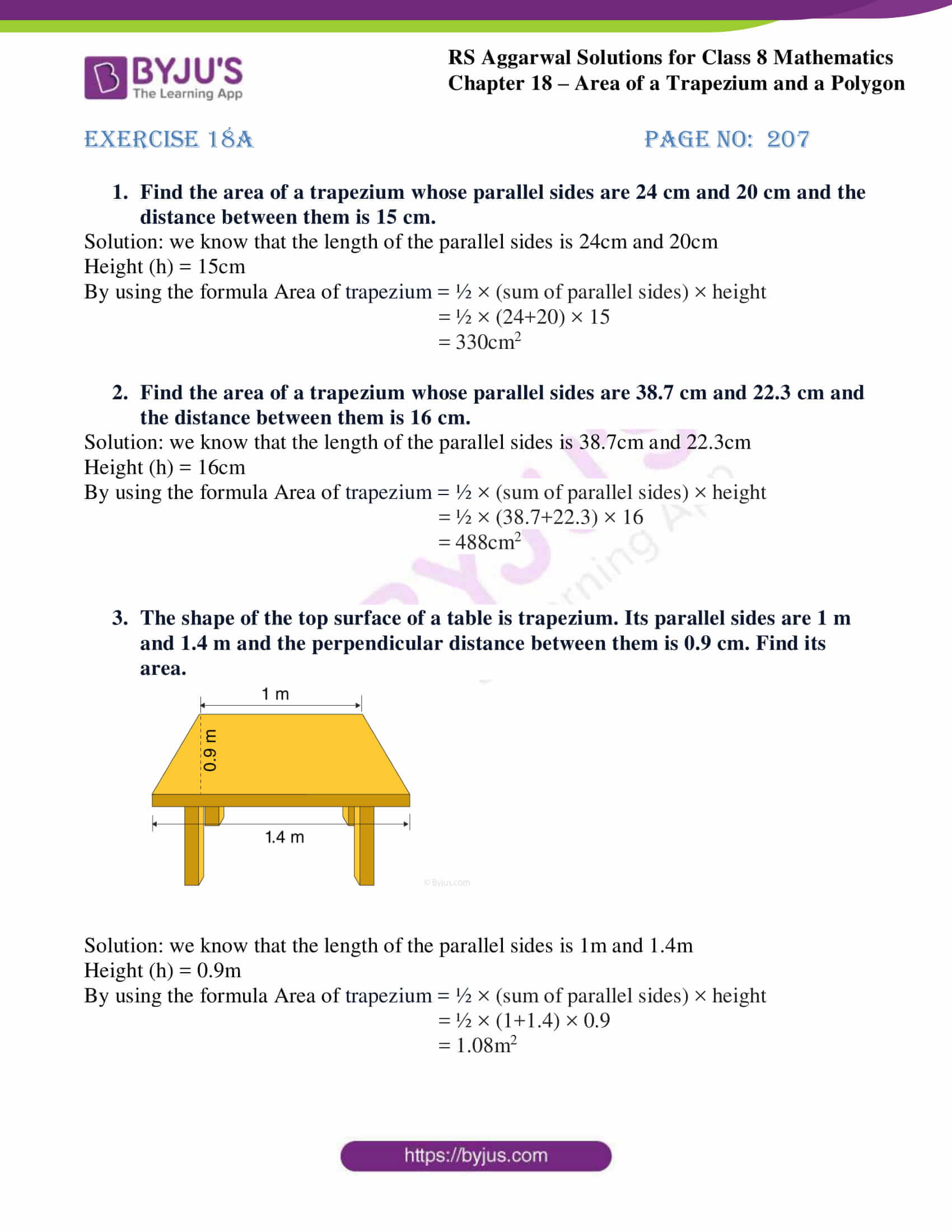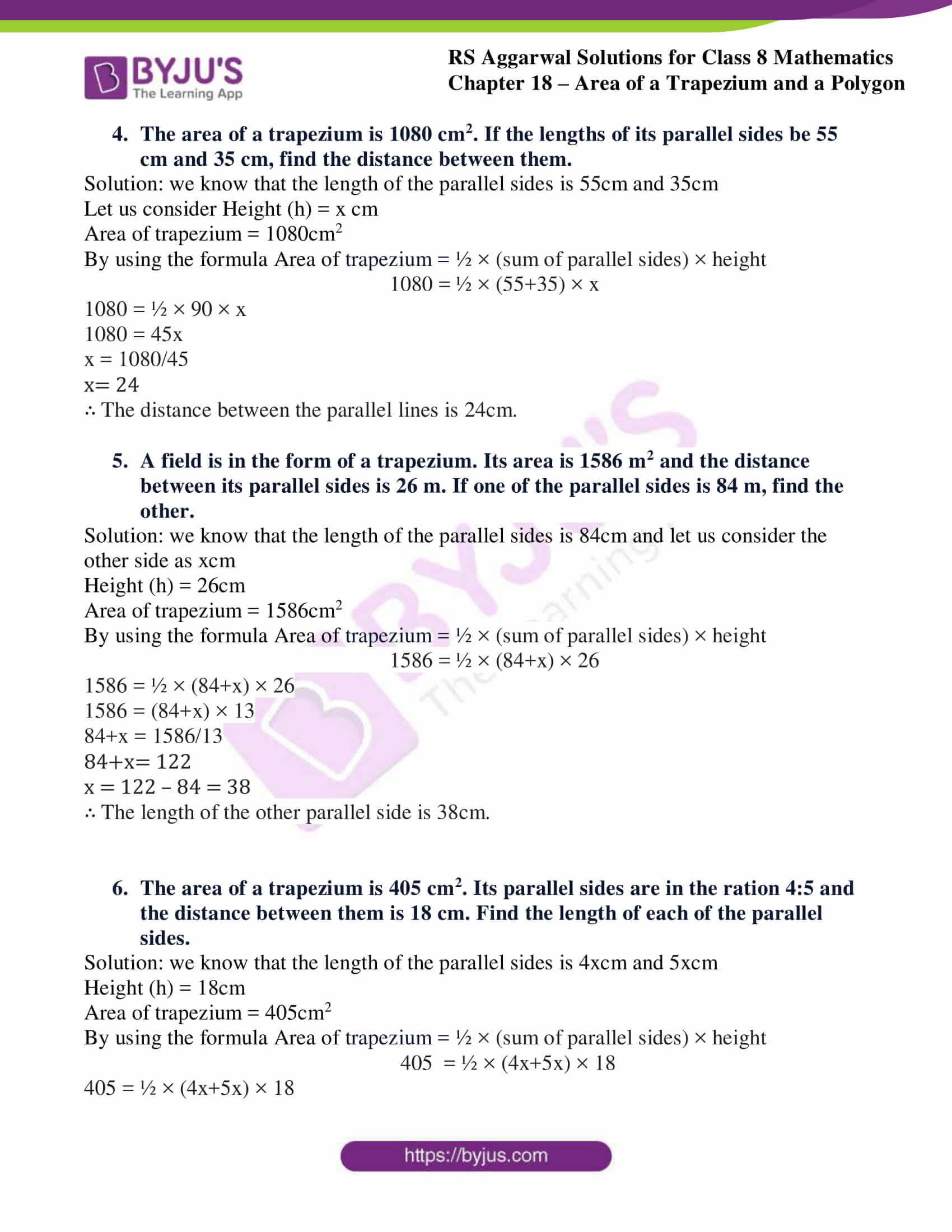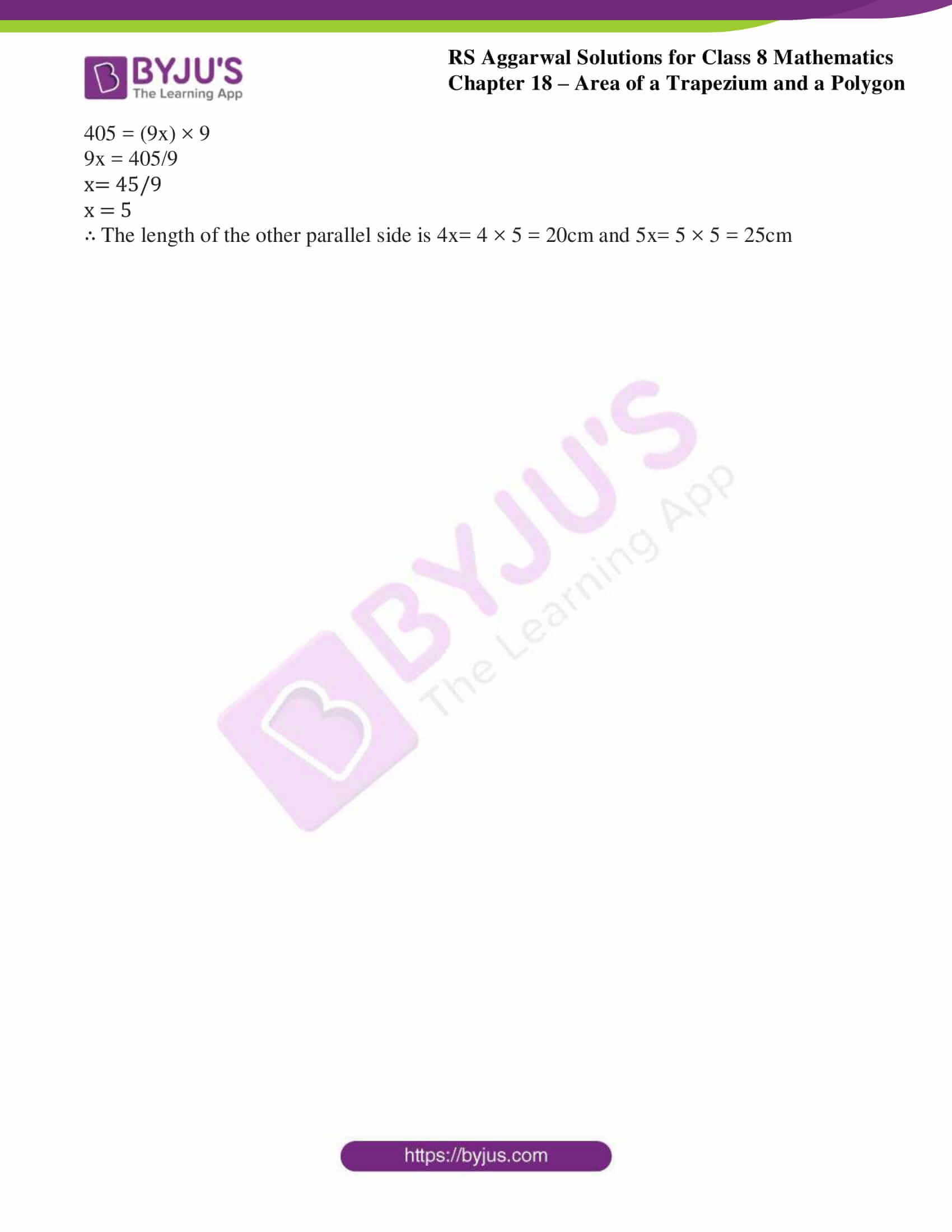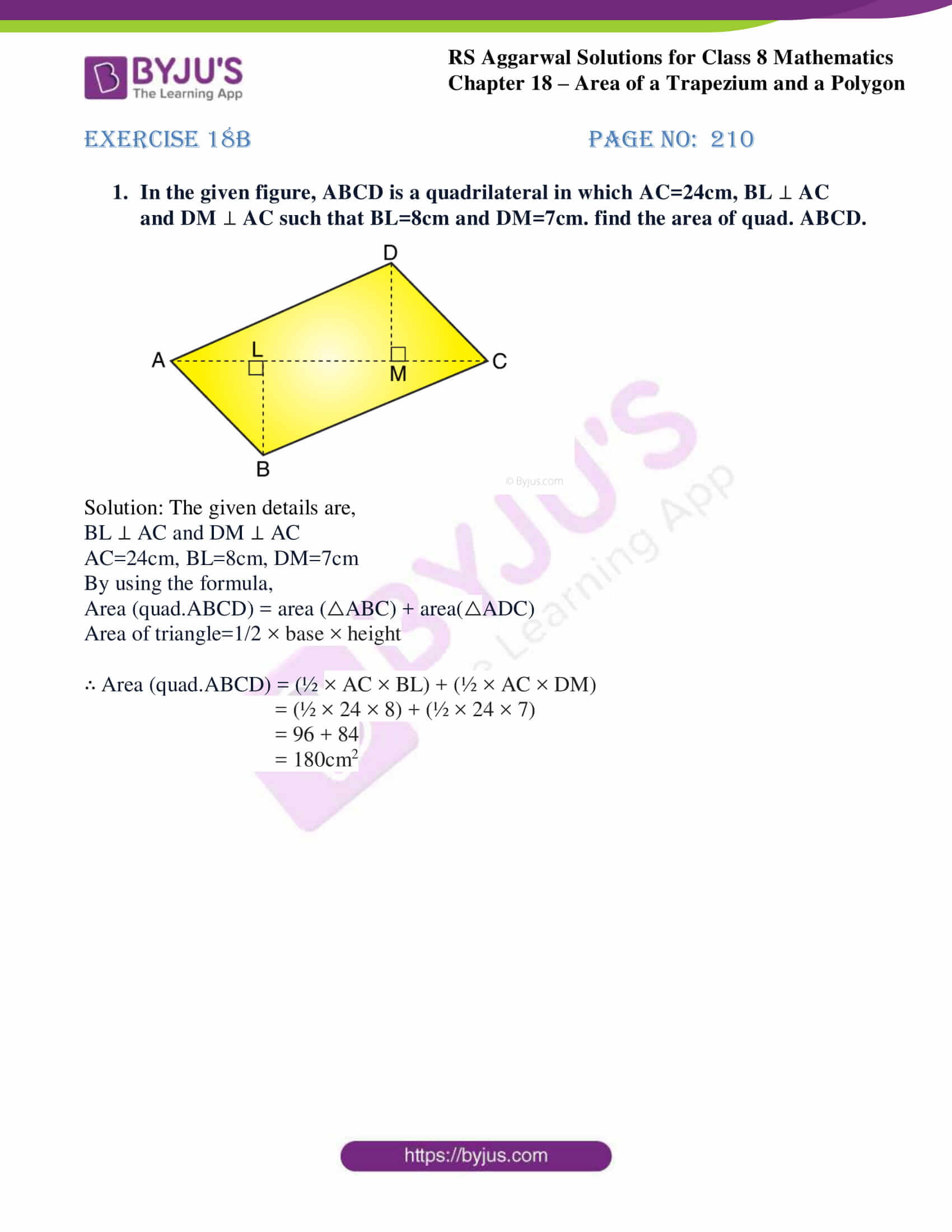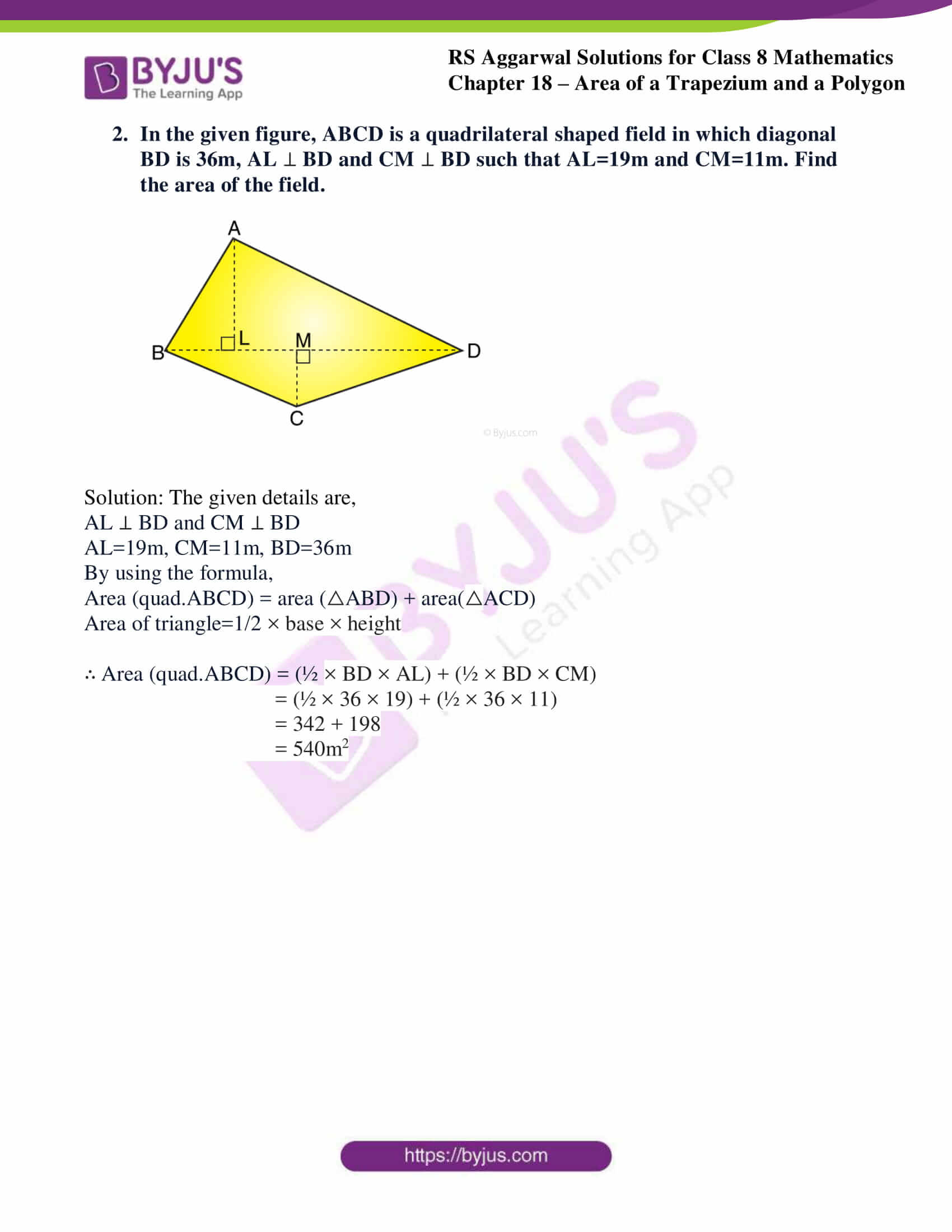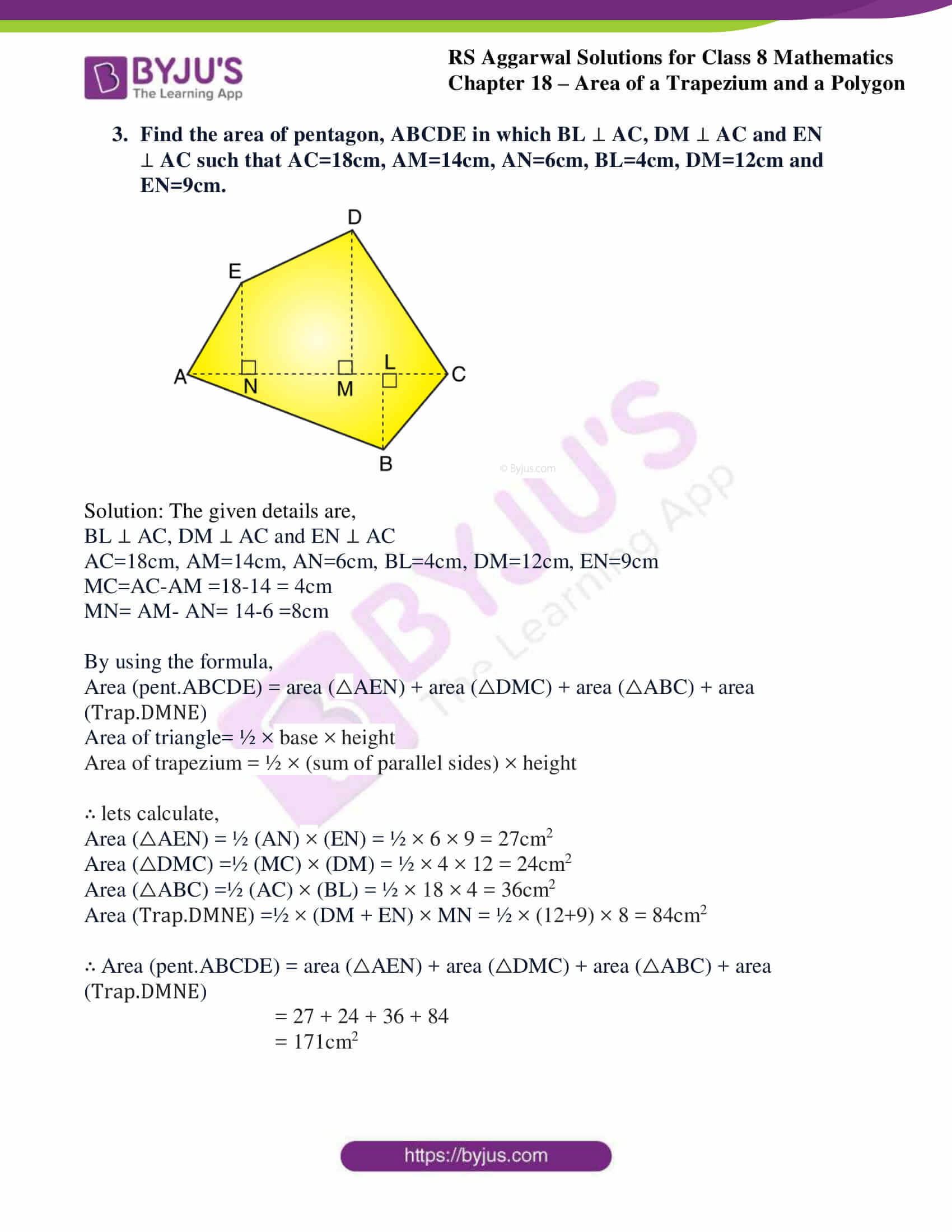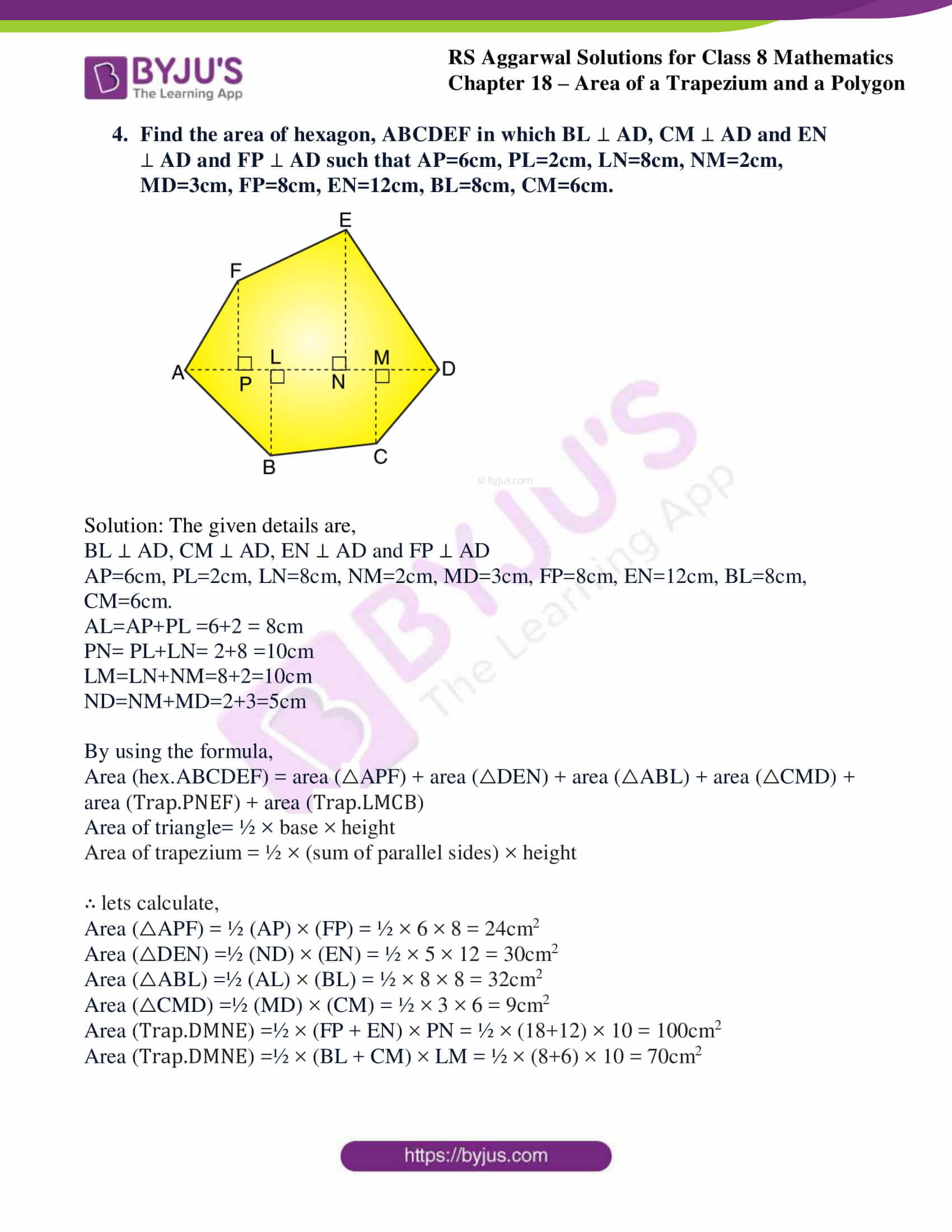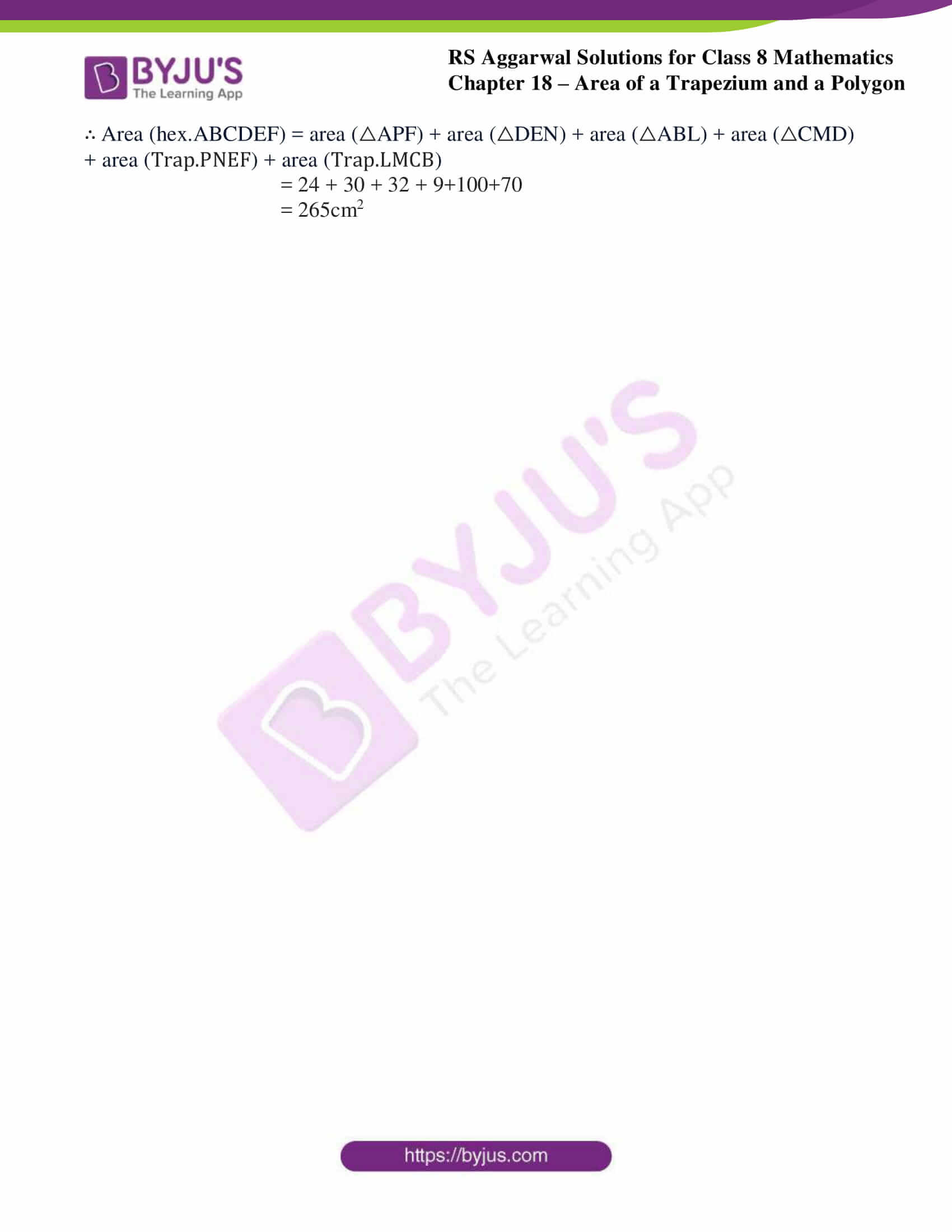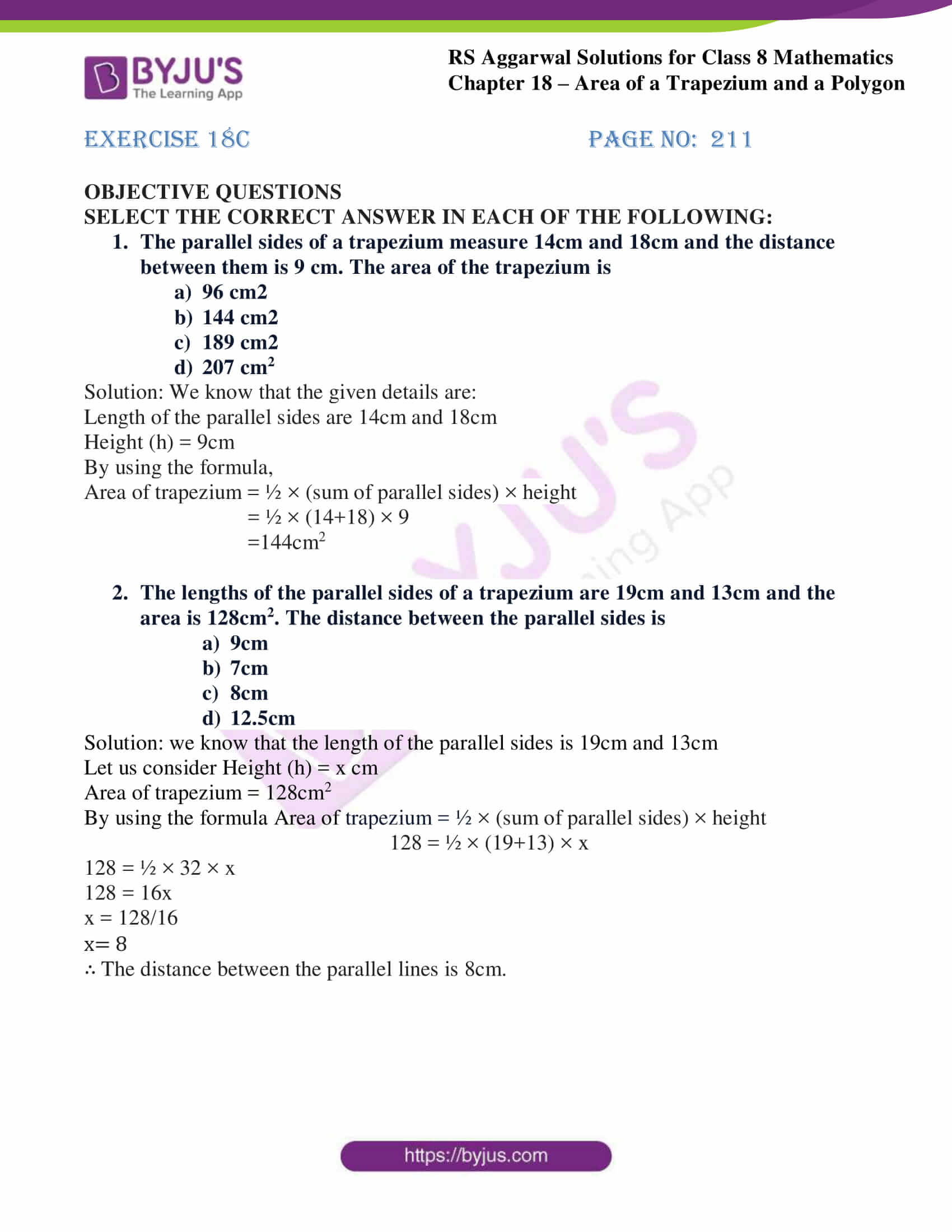## Exercise 18A

1. Find the area of a trapezium whose parallel sides are 24 cm and 20 cm and the distance between them is 15 cm.

Solution: we know that the length of the parallel sides is 24cm and 20cm

Height (h) = 15cm

By using the formula Area of trapezium = ½ × (sum of parallel sides) × height

= ½ × (24+20) × 15

= 330cm2

2. Find the area of a trapezium whose parallel sides are 38.7 cm and 22.3 cm and the distance between them is 16 cm.

Solution: we know that the length of the parallel sides is 38.7cm and 22.3cm

Height (h) = 16cm

By using the formula Area of trapezium = ½ × (sum of parallel sides) × height

= ½ × (38.7+22.3) × 16

= 488cm2

3. The shape of the top surface of a table is trapezium. Its parallel sides are 1 m and 1.4 m and the perpendicular distance between them is 0.9 cm. Find its area.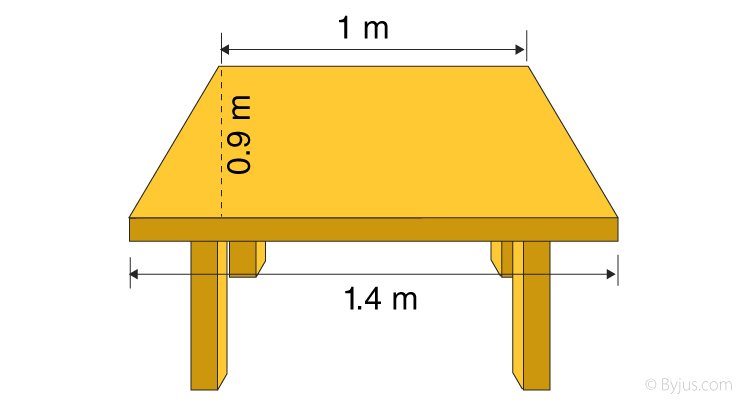Solution: we know that the length of the parallel sides is 1m and 1.4m

Height (h) = 0.9m

By using the formula Area of trapezium = ½ × (sum of parallel sides) × height

= ½ × (1+1.4) × 0.9

= 1.08m2

4. The area of a trapezium is 1080 cm2. If the lengths of its parallel sides be 55 cm and 35 cm, find the distance between them.

Solution: we know that the length of the parallel sides is 55cm and 35cm

Let us consider Height (h) = x cm

Area of trapezium = 1080cm2

By using the formula Area of trapezium = ½ × (sum of parallel sides) × height

1080 = ½ × (55+35) × x

1080 = ½ × 90 × x

1080 = 45x

x = 1080/45

x= 24

∴ The distance between the parallel lines is 24cm.

5. A field is in the form of a trapezium. Its area is 1586 m2 and the distance between its parallel sides is 26 m. If one of the parallel sides is 84 m, find the other.

Solution: we know that the length of the parallel sides is 84cm and let us consider the other side as xcm

Height (h) = 26cm

Area of trapezium = 1586cm2

By using the formula Area of trapezium = ½ × (sum of parallel sides) × height

1586 = ½ × (84+x) × 26

1586 = ½ × (84+x) × 26

1586 = (84+x) × 13

84+x = 1586/13

84+x= 122

x = 122 – 84 = 38

∴ The length of the other parallel side is 38cm.

6. The area of a trapezium is 405 cm2. Its parallel sides are in the ration 4:5 and the distance between them is 18 cm. Find the length of each of the parallel sides.

Solution: we know that the length of the parallel sides is 4xcm and 5xcm

Height (h) = 18cm

Area of trapezium = 405cm2

By using the formula Area of trapezium = ½ × (sum of parallel sides) × height

405 = ½ × (4x+5x) × 18

405 = ½ × (4x+5x) × 18

405 = (9x) × 9

9x = 405/9

x= 45/9

x = 5

∴ The length of the other parallel side is 4x= 4 × 5 = 20cm and 5x= 5 × 5 = 25cm

## Exercise 18B

1. In the given figure, ABCD is a quadrilateral in which AC=24cm, BL  AC and DM  AC such that BL=8cm and DM=7cm. find the area of quad. ABCD.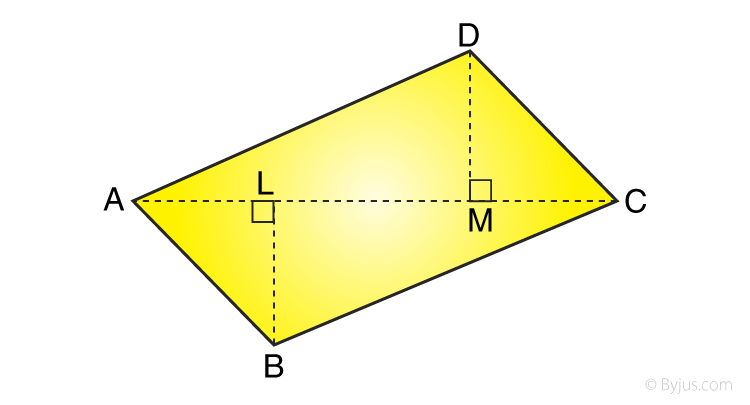Solution: The given details are,

BL ⊥ AC and DM ⊥ AC

AC=24cm, BL=8cm, DM=7cm

By using the formula,

Area of triangle=1/2 × base × height

∴ Area (quad.ABCD) = (½ × AC × BL) + (½ × AC × DM)

= (½ × 24 × 8) + (½ × 24 × 7)

= 96 + 84

= 180cm2

2. In the given figure, ABCD is a quadrilateral shaped field in which diagonal BD is 36m, AL  BD and CM  BD such that AL=19m and CM=11m. Find the area of the field.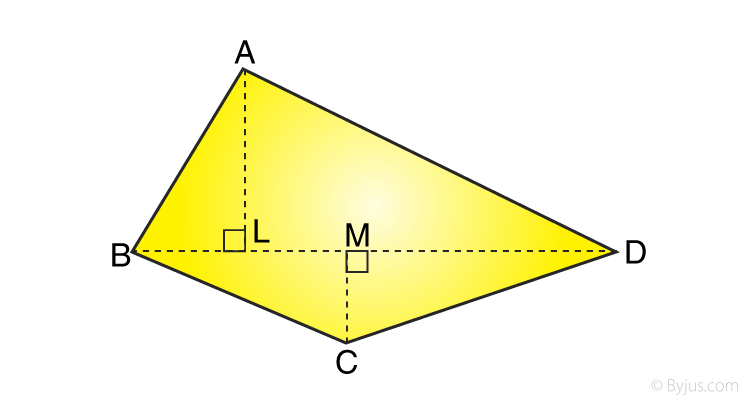Solution: The given details are,

AL ⊥ BD and CM ⊥ BD

AL=19m, CM=11m, BD=36m

By using the formula,

Area (quad.ABCD) = area (△ABD) + area(△ACD)

Area of triangle=1/2 × base × height

∴ Area (quad.ABCD) = (½ × BD × AL) + (½ × BD × CM)

= (½ × 36 × 19) + (½ × 36 × 11)

= 342 + 198

= 540m2

3. Find the area of pentagon, ABCDE in which BL  AC, DM  AC and EN  AC such that AC=18cm, AM=14cm, AN=6cm, BL=4cm, DM=12cm and EN=9cm.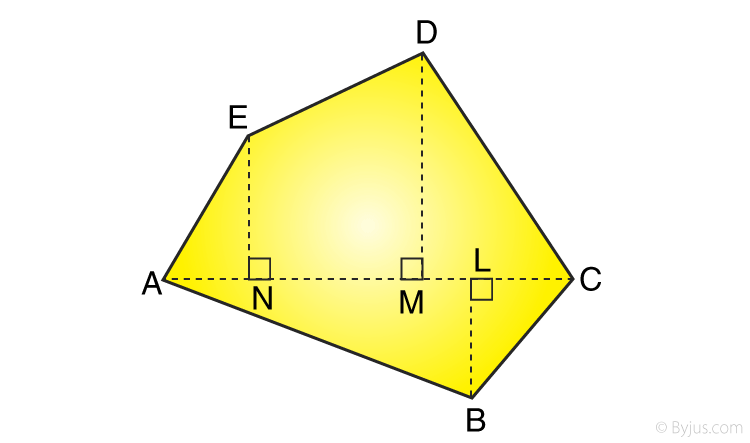Solution: The given details are,

BL ⊥ AC, DM ⊥ AC and EN ⊥ AC

AC=18cm, AM=14cm, AN=6cm, BL=4cm, DM=12cm, EN=9cm

MC=AC-AM =18-14 = 4cm

MN= AM- AN= 14-6 =8cm

By using the formula,

Area (pent.ABCDE) = area (△AEN) + area (△DMC) + area (△ABC) + area (Trap.DMNE)

Area of triangle= ½ × base × height

Area of trapezium = ½ × (sum of parallel sides) × height

∴ lets calculate,

Area (△AEN) = ½ (AN) × (EN) = ½ × 6 × 9 = 27cm2

Area (△DMC) =½ (MC) × (DM) = ½ × 4 × 12 = 24cm2

Area (△ABC) =½ (AC) × (BL) = ½ × 18 × 4 = 36cm2

Area (Trap.DMNE) =½ × (DM + EN) × MN = ½ × (12+9) × 8 = 84cm2

∴ Area (pent.ABCDE) = area (△AEN) + area (△DMC) + area (△ABC) + area (Trap.DMNE)

= 27 + 24 + 36 + 84

= 171cm2

4. Find the area of hexagon, ABCDEF in which BL  AD, CM  AD and EN  AD and FP  AD such that AP=6cm, PL=2cm, LN=8cm, NM=2cm, MD=3cm, FP=8cm, EN=12cm, BL=8cm, CM=6cm.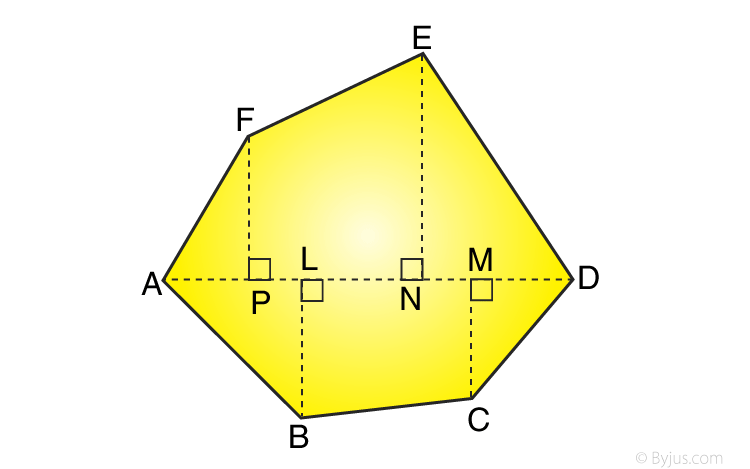Solution: The given details are,

AP=6cm, PL=2cm, LN=8cm, NM=2cm, MD=3cm, FP=8cm, EN=12cm, BL=8cm, CM=6cm.

AL=AP+PL =6+2 = 8cm

PN= PL+LN= 2+8 =10cm

LM=LN+NM=8+2=10cm

ND=NM+MD=2+3=5cm

By using the formula,

Area (hex.ABCDEF) = area (△APF) + area (△DEN) + area (△ABL) + area (△CMD) + area (Trap.PNEF) + area (Trap.LMCB)

Area of triangle= ½ × base × height

Area of trapezium = ½ × (sum of parallel sides) × height

∴ lets calculate,

Area (△APF) = ½ (AP) × (FP) = ½ × 6 × 8 = 24cm2

Area (△DEN) =½ (ND) × (EN) = ½ × 5 × 12 = 30cm2

Area (△ABL) =½ (AL) × (BL) = ½ × 8 × 8 = 32cm2

Area (△CMD) =½ (MD) × (CM) = ½ × 3 × 6 = 9cm2

Area (Trap.DMNE) =½ × (FP + EN) × PN = ½ × (18+12) × 10 = 100cm2

Area (Trap.DMNE) =½ × (BL + CM) × LM = ½ × (8+6) × 10 = 70cm2

∴ Area (hex.ABCDEF) = area (△APF) + area (△DEN) + area (△ABL) + area (△CMD) + area (Trap.PNEF) + area (Trap.LMCB)

= 24 + 30 + 32 + 9+100+70

= 265cm2

## Exercise 18C

SELECT THE CORRECT ANSWER IN EACH OF THE FOLLOWING:

1. The parallel sides of a trapezium measure 14cm and 18cm and the distance between them is 9 cm. The area of the trapezium is
96 cm2
144 cm2
189 cm2
207 cm2

Solution: We know that the given details are:

Length of the parallel sides are 14cm and 18cm

Height (h) = 9cm

By using the formula,

Area of trapezium = ½ × (sum of parallel sides) × height

= ½ × (14+18) × 9

=144cm2

2. The lengths of the parallel sides of a trapezium are 19cm and 13cm and the area is 128cm2. The distance between the parallel sides is
9cm
7cm
8cm
12.5cm

Solution: we know that the length of the parallel sides is 19cm and 13cm

Let us consider Height (h) = x cm

Area of trapezium = 128cm2

By using the formula Area of trapezium = ½ × (sum of parallel sides) × height

128 = ½ × (19+13) × x

128 = ½ × 32 × x

128 = 16x

x = 128/16

x= 8

∴ The distance between the parallel lines is 8cm.

## RS Aggarwal Solutions for Class 8 Maths Chapter 18 – Area of a Trapezium and a Polygon

Chapter 18- Area of a Trapezium and a Polygon contains 3 exercises and the RS Aggarwal solutions present on this page provide the solutions for the questions present in each exercise. Now, let us have a look at the concepts discussed in this chapter.

• Area of a Trapezium.
• Area of a Polygon.

Exercise 18A

Exercise 18B

Exercise 18C

### Chapter Brief of RS Aggarwal Solutions for Class 8 Maths Chapter 18 – Area of a Trapezium and a Polygon

RS Aggarwal Solutions for Class 8 Maths Chapter 18 – Area of a Trapezium and a Polygon, ensures that the students are thorough and familiar with the concepts. Regular revision of important concepts and formulas over time to time is the best way to strengthen your concepts.

As the Chapter is about Area of a Trapezium and a Polygon, here we calculate and find the area of a Trapezium, area of a Polygon using their respective formulas. In the RS Aggarwal Solutions book, many such exercise problems are given which enhances familiarity with the concepts.